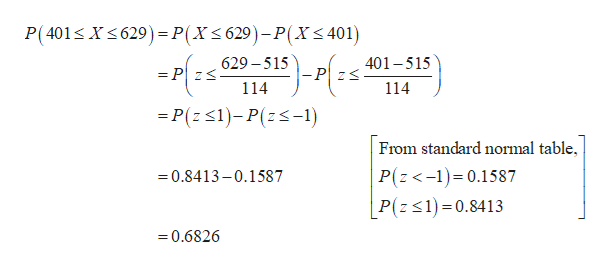# SAT Math scores have a bell-shaped distribution with a mean of 515 and a standard deviation of 114. a) What percentage of SAT scores is between 401 and 629b) What percentage of SAT score is less than 401 or greater than 629?

Question
63 views

SAT Math scores have a bell-shaped distribution with a mean of 515 and a standard deviation of 114.

a) What percentage of SAT scores is between 401 and 629

b) What percentage of SAT score is less than 401 or greater than 629?

check_circle

Step 1

a.

The percentage of SAT scores is between 401 and 629 is 0.6826 and it is calculated below:

From the given information, let us define the rand...help_outlineImage TranscriptioncloseP(401 Xs629) P(X< 629)-P(X< 401) 401-515 629-515 = P zS - PzS _ 114 114 =P(z 1)-P(z-1) From standard normal table, P(z <-1)0.1587 0.8413-0.1587 P(z1)=0.8413 0.6826 fullscreen

### Want to see the full answer?

See Solution

#### Want to see this answer and more?

Solutions are written by subject experts who are available 24/7. Questions are typically answered within 1 hour.*

See Solution
*Response times may vary by subject and question.
Tagged in

### Other